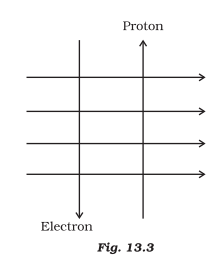# A uniform magnetic field exists in the plane of paper pointing from left to right as shown in the figure. In the field an electron and a proton move as shown. The electron and the proton experience (a) forces both pointing into the plane of paper (b) forces both pointing out of the plane of paper (c) forces pointing into the plane of paper and out of the plane of paper, respectively (d) force pointing opposite and along the direction of the uniform magnetic field respectively(a) forces both pointing into the plane of the paper

### Explanation

For the given figure, the proton and electron are moving in opposite directions to each other and is perpendicular to the direction of magnetic field. Now, we know that the direction of current is taken opposite to the direction of motion of electron. So, both electron and proton have current in same direction. Therefore, the forces acting on them are given by Fleming s left hand rule and they are pointing into the plane of the paper.(0)(0)